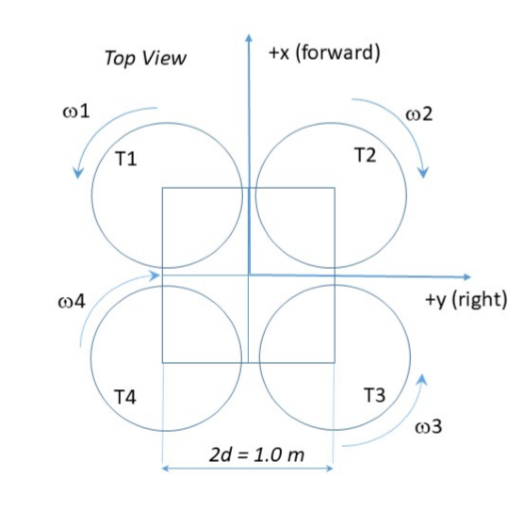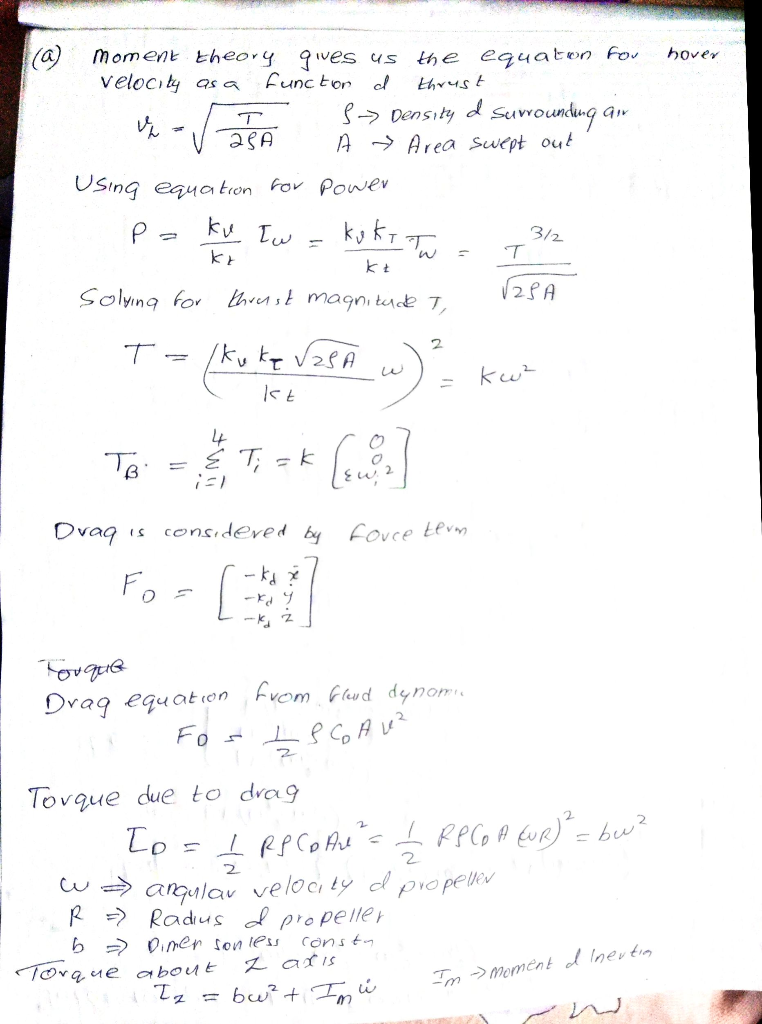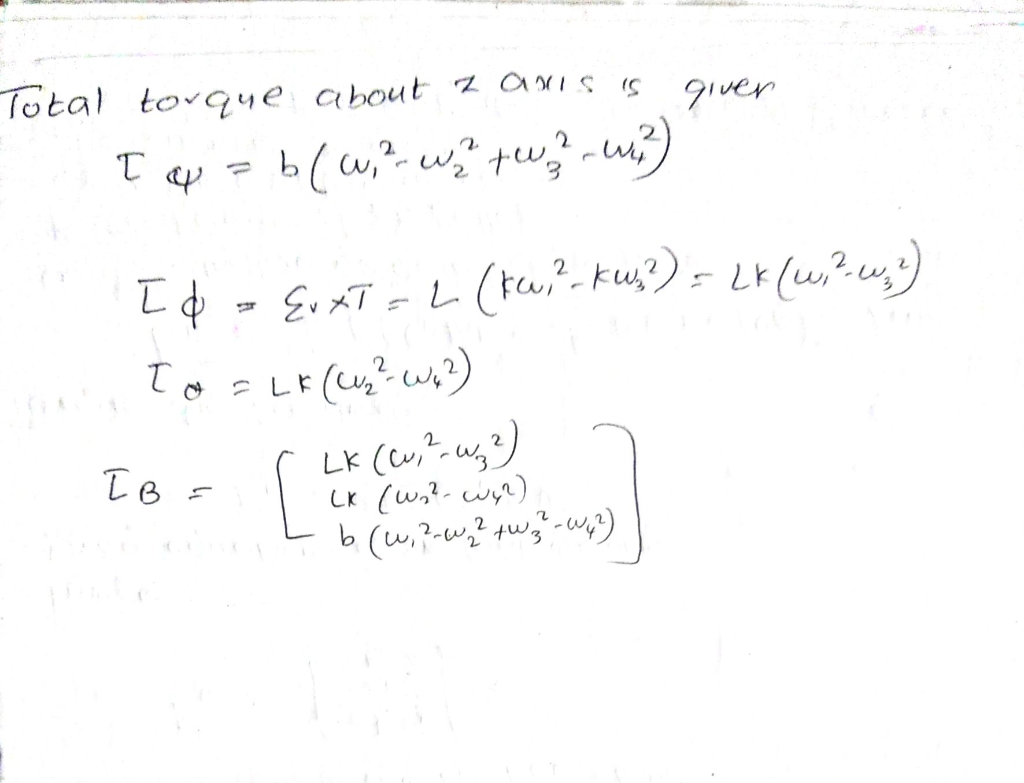# 1) A quadrotor ﬂies by applying four thrust vectors [T1,T2,T3,T4] to achieve total force f and...

1) A quadrotor ﬂies by applying four thrust vectors [T1,T2,T3,T4] to achieve total force f and moments [Mx,My,Mz]. Assume all four quadrotor propellers lie in a common plane, each motor’s thrust acts perpendicular to the propeller disk, and that adjacent quadrotor motor shafts are separated as shown in the below ﬁgure. Assume the axis system deﬁned in the below ﬁgure for all parts of this question, and that the center of gravity of the quadrotor is at the body midpoint ((xb,yb) = (0,0)) equidistant from all thrusters.

Suppose a quadrotor with mass m = 5kg has coeﬃcient of thrust CT = 1.4E −5 and coeﬃcient of moment CM = 3E −6. Note that the CT and CM coeﬃcients have units of kg ·m and kg ·m2, respectively, given motor speeds ωi in rad/sec.

a) What four motor speeds ωi are required for the quadrotor to hover?

b) Suppose the quadrotor experiences a drag force D = cDQV linear in horizontal speed V with quadrotor coeﬃcient of drag cDQ = 0.1kg/s. What pitch angle θ will allow the quadrotor to ﬂy forward in steady ﬂight at 5m/s?~~~ This quadrotor is in X-Configuration

Top View +x (forward) 01 02 T2 004 +y (right) 03 2d = 1.0 m#### Earn Coin

Coins can be redeemed for fabulous gifts.